# Zbornik Veleučilišta u Rijeci,Vol. 7 No. 1, 2019.

Izvorni znanstveni članak
https://doi.org/10.31784/zvr.7.1.11

NUMERICAL ANALYSIS OF THE FUEL SPRAY PACKAGES PENETRATION AND GAS INFLOW FROM QUASI-DIMENSIONAL DI DIESEL ENGINE NUMERICAL MODEL

Vedran Mrzljakorcid.org/0000-0003-0323-2600 ; University of Rijeka, Faculty of Engineering, Department of Thermodynamics and Energy Engineering, Rijeka, Croatia
Božica Žarković ; University of Rijeka, Faculty of Engineering, Rijeka, Croatia

 Puni tekst: engleski, pdf (2 MB) str. 335-357 preuzimanja: 151* citiraj APA 6th EditionMrzljak, V. i Žarković, B. (2019). NUMERICAL ANALYSIS OF THE FUEL SPRAY PACKAGES PENETRATION AND GAS INFLOW FROM QUASI-DIMENSIONAL DI DIESEL ENGINE NUMERICAL MODEL . Zbornik Veleučilišta u Rijeci, 7 (1), 335-357. https://doi.org/10.31784/zvr.7.1.11 MLA 8th EditionMrzljak, Vedran i Božica Žarković. "NUMERICAL ANALYSIS OF THE FUEL SPRAY PACKAGES PENETRATION AND GAS INFLOW FROM QUASI-DIMENSIONAL DI DIESEL ENGINE NUMERICAL MODEL ." Zbornik Veleučilišta u Rijeci, vol. 7, br. 1, 2019, str. 335-357. https://doi.org/10.31784/zvr.7.1.11. Citirano 25.02.2020. Chicago 17th EditionMrzljak, Vedran i Božica Žarković. "NUMERICAL ANALYSIS OF THE FUEL SPRAY PACKAGES PENETRATION AND GAS INFLOW FROM QUASI-DIMENSIONAL DI DIESEL ENGINE NUMERICAL MODEL ." Zbornik Veleučilišta u Rijeci 7, br. 1 (2019): 335-357. https://doi.org/10.31784/zvr.7.1.11 HarvardMrzljak, V., i Žarković, B. (2019). 'NUMERICAL ANALYSIS OF THE FUEL SPRAY PACKAGES PENETRATION AND GAS INFLOW FROM QUASI-DIMENSIONAL DI DIESEL ENGINE NUMERICAL MODEL ', Zbornik Veleučilišta u Rijeci, 7(1), str. 335-357. https://doi.org/10.31784/zvr.7.1.11 VancouverMrzljak V, Žarković B. NUMERICAL ANALYSIS OF THE FUEL SPRAY PACKAGES PENETRATION AND GAS INFLOW FROM QUASI-DIMENSIONAL DI DIESEL ENGINE NUMERICAL MODEL . Zbornik Veleučilišta u Rijeci [Internet]. 2019 [pristupljeno 25.02.2020.];7(1):335-357. https://doi.org/10.31784/zvr.7.1.11 IEEEV. Mrzljak i B. Žarković, "NUMERICAL ANALYSIS OF THE FUEL SPRAY PACKAGES PENETRATION AND GAS INFLOW FROM QUASI-DIMENSIONAL DI DIESEL ENGINE NUMERICAL MODEL ", Zbornik Veleučilišta u Rijeci, vol.7, br. 1, str. 335-357, 2019. [Online]. https://doi.org/10.31784/zvr.7.1.11

Sažetak
The paper presents two components of developed quasi-dimensional numerical model: spray package penetration and gas inflow from the zone without combustion into the spray packages. Correction of spray package penetration along the fuel spray radial axis is presented and described numerically. Numerical model simulation is validated in several measured operating points of direct injection diesel engine, after which the simulation results are presented. According to numerical model settings, each fuel spray was divided into packages (control volumes). In the same time interval, the shortest penetration was obtained for spray packages located at the fuel spray edge. Before reaching the break-up time spray package penetration was linear and after reaching the break-up time, package penetration had a curved form. Later injected spray packages quickly reached and surpassed earlier injected spray packages because earlier injected spray packages hit “gas wall” in the engine cylinder and they were “braked” with surrounding gas in comparison to packages injected later. Gas inflow from the zone without combustion into the spray packages is the most intensive for spray packages positioned at the fuel spray edge. The simulation shows that the inflow mass from the zone without combustion increases for spray packages injected later.

Hrčak ID: 219996

▼ Article Information

##### 1. INTRODUCTION

Before the development of quasi-dimensional numerical models, two different types of numerical models for internal combustion engine process simulations were developed. The first ones were Zero-dimensional (0D) models and the second ones were Computational Fluid Dynamics (CFD) numerical models. 0D numerical models assume a homogeneous mixture of gases in the engine cylinder and they do not have possibilities in predicting engine emissions such as NOx, CHx and particles (Medica, 1988) , (Guan et al., 2015) . The speed and intensity of chemical reactions responsible for pollutant formation are highly sensitive to local temperature field and local species concentrations. When assuming a homogeneous mixture of gases in the engine cylinder, it is practically impossible to obtain cylinder local temperature fields and species concentrations, necessary for accurate emissions predictions. The most detailed internal combustion engine simulations can be obtained with CFD numerical models. The downside of such simulations is an extremely long time of calculation (Meloni, Naso, 2013) , they are not accessible for the whole engine simulations, i.e. simulations are usually limited to just one engine cylinder (Ramesh, Mallikarjuna, 2017) ,

(Huang et al., 2016) and in most cases some of the input data needs to be assumed (Senčić, 2010) .

Quasi-dimensional models were developed as a compromise between 0D and CFD models. The development of such models started with the initial division of the space inside the cylinder into two or more zones - one or more zones for fuel spray and one fresh mixture zone (Ishida et al., 1994) , (Rakopoulos et al., 2004) . This kind of models enabled a rude prediction in the engine emissions, but not a detailed one. The quasi-dimensional model presented in this paper begins at the start of fuel injection. Boundary conditions of QD model are geometrical parameters of fuel injector and cylinder, fuel current pressure and thermodynamic properties of gas in the cylinder at the start of fuel injection. The fuel flow contraction in the nozzle is calculated by using equations from Von Kuensberg Sarre et al. (Von Kuensberg Sarre et al., 1999) . Liquid fuel spray decomposition and calculation of droplets number and diameter is performed by using equations from Mayer, Branam (Mayer, Branam, 2004) . Liquid fuel energy conservation equation is used to monitor the liquid fuel temperature - a basic parameter for the fuel evaporation. The injected fuel spray is divided into spray packages (volumes) and new spray packages are added as injection continues. Such set of spray packages accompanies each fuel spray, Figure 1. Outside the fuel sprays is a large zone without combustion - ZWC (Hiroyasu et al., 2002) , (Mrzljak et al., 2017) . If injectors have more, mutually different nozzles, separate volumes are created for each of the fuel sprays.

Figure 1. Cylinder section with fuel injector and three fuel sprays divided into packages (control volumes)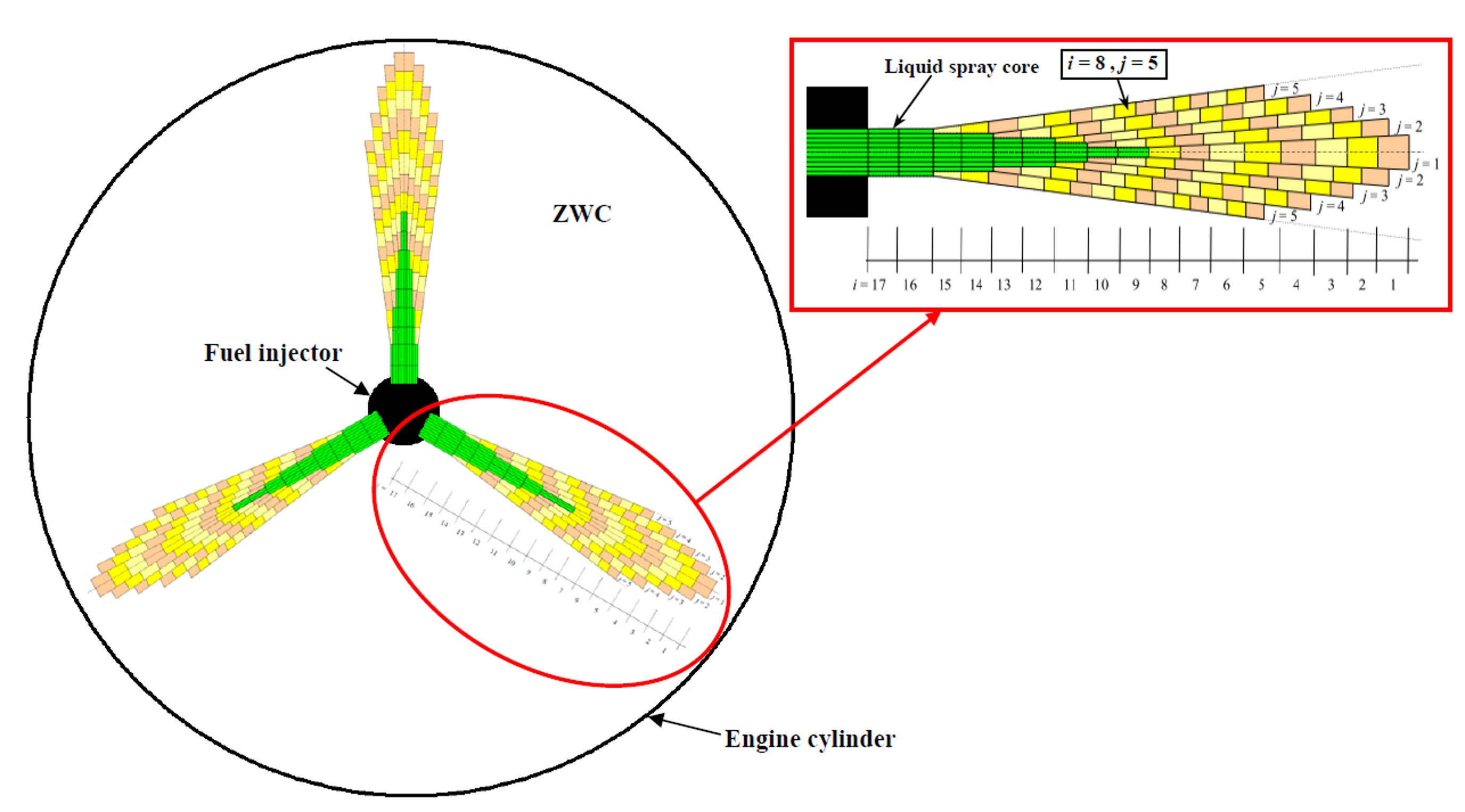Source: Authors’ original work

The basic assumption of such a model states that between fuel spray packages no exchange of mass or energy is allowed (Mrzljak et al., 2016a) . The only allowed mass exchange is the gas inflow from the zone without combustion (ZWC) into spray packages (Hiroyasu, Arai, 1990) , when the necessary conditions are fulfilled in each spray package. According to Figure 1, with the indexes which are related to each spray package, (i = axial index, j = radial index), it was necessary to use an additional index k for each fuel spray when the fuel sprays are not equal. In case that fuel sprays are equal, one spray is calculated and the results are transferred to the rest of identical fuel sprays. The axial (spray longitudinal) index i depends on the amount of fuel injected into the engine cylinder during each cylinder process. The radial index j can be chosen arbitrarily. In the developed QD numerical model, the fuel spray is divided into 5 radial zones (packages) per one axial row. The same division can be found in other scientific papers which investigate the same topic (Hiroyasu et al., 2002) , (Stiesch, 1999) , (Mrzljak et al., 2015) .

Nowadays, researchers are intensively involved in implementing the combustion of alternative fuels in existing internal combustion engines. In that way, engine emissions can be greatly reduced and some engine operating parameters can be improved. For example, the biogas usage in the internal combustion engine can reduce NOx emissions by more than 90% due the low combustion temperatures (Jung et al., 2015) . The biodiesels have lesser ignition delay and lower soot emissions in comparison with pure diesel (Rajak et al., 2018) . The oxygen content of biodiesel fuel is beneficial for improving the in-cylinder combustion, especially at high engine loads (Zhang et al., 2018) . The usage of hydrogen as a fuel in internal combustion engine can significantly improve the mixture uniformity in a cylinder under different engine speeds, equivalent ratios and nozzle diameters (Yang et al., 2018) . Liu presented an experimental investigation of performance, combustion and emission characteristics of a common-rail diesel engine fuelled with polyoxymethylene dimethyl ethers-diesel blends (Liu et al., 2017) . Compared with pure diesel fuel, the smoke emissions at full load are reduced up to 47.6%, while the concentration of particles decreases up to 43.79% with used blends. K. Lee investigated performance and emission characteristics of a DI diesel engine which operates with blended fuel (Lee, Kim, 2017) . The obtained results show that blended fuel produced lower hydrocarbons (HC), carbon monoxide (CO) and particulate matter (PM) in comparison with pure diesel. Nitrogen oxides (NOx) from the blended fuel were higher than that of diesel due to the shorter ignition delay and high oxygen content. Jamrozik investigated the effect of the alcohol content in the fuel mixture on the performance and emissions of a direct injection diesel engine (Jamrozik, 2017) . The methanol content increase in the fuel mixture led to a substantial reduction in carbon monoxide, hydrocarbons and carbon dioxide emissions. Hoseini investigated biodiesel fuel blends (blends with Salvia Macrosiphon oil) for the use in diesel engines (Hoseini et al., 2017) . With these blends, CO and HC were reduced by up to 25% and 31.82%, power and torque increased up to 18% and 15.8%, while specific fuel consumption decreased by 4.6% in comparison with pure diesel. Many other researchers investigated this field. A complete review for green fuels as alternative fuels for diesel engines is presented by Othman (Othman et al., 2017) while the review of performance, combustion and emission characteristics of biodiesel fuelled diesel engines is presented by Tamilselvan (Tamilselvan et al., 2017) . By implementing the characteristics and properties of alternative fuels into a program code, along with some model modifications, numerical simulations of diesel engines running with fuel blends or pure alternative fuels can be obtained with the presented quasi-dimensional model.

##### 2. NUMERICAL MODEL OF SPRAY PACKAGES PENETRATION AND GAS INFLOW

2.1 Fuel spray packages penetration

The term “fuel spray package penetration” implies the distance between the center of gravity of each fuel spray package and the fuel injector nozzle. This is the only parameter in QD numerical models that directly shows the intermittent (transient) fuel spray behaviour in direct injection diesel engines. The position of the fuel spray head (the most prominent package) can usually be determined by photographing of the fuel spray (Wang et al., 2019) . Experiments have shown that there are two different phases in the motion of each fuel spray package (Cvetić, 2000) , (Mrzljak et al., 2016a) . In the first phase, the rate of package penetration is practically constant and then in the second phase it progressively decreases. Both phases show linear dependence between the time logarithm and the package penetration logarithm.

The first parameter which must be defined in order to calculate spray package penetration is a characteristic break-up time. When this time expires, the gas from the ZWC begins to inflow into the spray package, after which liquid fuel is falling apart into the droplets. This time is calculated for each spray package independently because gas inflow from the ZWC starts earlier into the packages on the fuel spray edges in comparison with the packages in the fuel spray core.

The break-up time for the packages in the fuel spray core is defined by the equation (Cvetić, 2000) :

(1)
${t}_{b}=B \cdot \frac{{\rho }_{f} \cdot d}{\left({\rho }_{g} \cdot \Delta p{\right)}^{0.5}}$

while for the fuel spray edges, the break-up time is defined in a way that it linearly decreases from the spray core (jmin=1) up to the spray edges (jmax=5), (Stiesch, 1999) :

(2)
${t}_{\text{b,j}}={t}_{b} \cdot \left(1-\frac{j-1}{{j}_{\text{max}}}\right)$

Numerous authors defined the coefficient B from the equation (1) as a constant (Cvetić, 2000) , (Stiesch, 2003) with value B = 28.65. However, if this coefficient is adopted as a constant for all packages, a specific problem occurs during the calculation of each spray package penetration. When calculating a package penetration, the package progresses linearly until the break-up time is reached. After that, with the assumption that the coefficient B is a constant, the package penetration begins to decrease (which would in practice mean that package, instead of further advancement, returns to the fuel injector nozzle) and after some time, package begins to move further towards the cylinder walls. In order to avoid such physically impossible effect, the coefficient B is calculated iteratively. The value of the coefficient B and therefore the package break-up time must be calculated individually for each package. In that way a continuous curves for fuel spray package penetration will be obtained, without unexpected effects.

The correction of the coefficient B is presented in (Mrzljak, 2015) . With a mathematical excerpt the value of coefficient B for the axial fuel spray axis (j = 1) equal to B = 28.802 is obtained. For the packages outside the core of the fuel spray (from j = 2 to j = 5), the coefficient B should be calculated according to the equation:

(3)
$B=28.802 \cdot \frac{{\left\{\mathit{exp} \left[-0.0386 \cdot {\left(j-1\right)}^{2}\right]\right\}}^{2}}{\left(1-\frac{j-1}{{j}_{\mathit{max}}}\right)}$

The equation (3) shows that the coefficient B increases with the increase of index j and at the same time the break-up time decreases. One indispensable parameter for defining spray package penetration is the fuel injection velocity vi which is defined by the expression:

(4)
${v}_{i}={C}_{d} \cdot \sqrt{\frac{2 \cdot \Delta p}{{\rho }_{f}}}$

The variable Cd is a constant and in Stiesch (Stiesch, 1999) its value is defined as Cd = 0.39. The spray package penetration is defined in dependence of the break-up time, according to the equation (2). The spray package penetration in time 0 < t < tb is defined according to Cvetić (Cvetić, 2000) with an equation:

(5)
$S={v}_{i} \cdot t={C}_{d} \cdot \sqrt{\frac{2 \cdot \Delta p}{{\rho }_{f}}} \cdot t$

while in time t>tb spray package penetration is defined as:

(6)
${S}_{1}=2 \cdot 1.48 \cdot \sqrt{\frac{\Delta p}{{\rho }_{g}}} \cdot \sqrt{d \cdot t} \cdot \mathit{exp} \left[-0.0386 \cdot {\left(j-1\right)}^{2}\right]$
(7)
${S}_{2}={S}_{1} \cdot {\left(1+\frac{\pi \cdot n \cdot {R}_{S} \cdot {S}_{1}}{30 \cdot {v}_{i}}\right)}^{-1}$
(8)
$S={S}_{2} \cdot \left(1+\sqrt{\frac{i-1}{{i}_{\mathit{max}}-1}} \cdot 0.2\right)$

In equation (6) expression $\mathit{exp}\left(-0.0386 \cdot {\left(j-1\right)}^{2}\right)$ represents a factor for spray package velocity correction. As break-up time is defined differently for packages in the fuel spray core in relation to the packages located on the fuel spray periphery, equations (1) and (2), the correction of the package velocity must be performed in the same way. The correction takes into account all the packages which are not located in the fuel spray core, the reduction of their velocities and penetrations, in comparison with the packages of the same axial index located in the spray core. Index j is a spray package radial index. The reason of velocity reduction for the packages at the fuel spray periphery is that in these packages, gas from the ZWC enters earlier than in the packages in the fuel spray core (gas inflow will occur earlier for the package closer to the ZWC). The gas inflow into the fuel spray package, which will happen after the break-up time expires, resulted in package velocity reduction. This characteristic is also confirmed by measurements of the visible fuel jet contour, where it can be observed that the fuel spray head had the highest velocity and the highest penetration, while all the other parts of the fuel spray have a lower velocity and thus a smaller penetration in the same time interval (Von Kuensberg Sarre et al., 1999) , (Baumgarten, 2006) .

In equation (7) expression ${\left(1+\frac{\pi \cdot n \cdot {R}_{S} \cdot {S}_{1}}{30 \cdot {v}_{i}}\right)}^{-1}$ represents the correction factor of the fuel spray penetration relative to the swirl which occurs in the engine cylinder. The condition in the cylinder, especially during the intake, is not calm because gas flows in the form of a swirl which has a great influence on the fuel spray. The swirl moves fuel spray and turns its head, what is taken into account with this expression.

The equation (8) presents the final expression for defining fuel spray package penetration after the break-up time has expired. The expression $\left(1+\sqrt{\frac{i-1}{{i}_{\mathit{max}}-1}} \cdot 0.2\right)$ takes into account the third, final correction for fuel spray penetration. The kinetic energy of fuel spray which enters into the engine cylinder is higher in comparison with the cylinder swirl for two orders of magnitude. As a consequence, the initial parts of the fuel spray are more “braked” with surrounding gas than those which are injected subsequently and which travel in the “shadow” of the front spray parts. For these reasons it is necessary to make this correction, which depends on the axial (longitudinal) fuel spray package position (index i). The fuel injection velocity is not corrected. Index imax represents the maximum number of axial spray packages. Therefore, for the calculation of each package penetration the injection duration must be known in order to determine the maximum axial index.

2.2 Gas inflow from the ZWC into the fuel spray packages

In order to form a fuel mixture which will burn in the engine cylinder, along with high enough temperature, two components are required: fuel vapor (which is obtained from the liquid fuel) and air. The term air in quasi-dimensional numerical model implies gas, which inflows from the zone without combustion (ZWC) into each individual fuel spray package (Figure 1). That gas consists mainly of fresh air with a small amount of combustion products from the previous process which have not been released from the cylinder during the exhaust. Therefore, when calculating the gas inflow into the fuel spray packages, the calculation cannot be obtained only with the air as ideal gas, the remaining combustion products from the previous process must also be taken into account. The definition of gas inflow into the fuel spray package is based on the equation for momentum preservation:

(9)
${m}_{\text{f,in}} \cdot {v}_{i}=\left({m}_{\text{f,in}}+{m}_{\text{gi}}\right) \cdot \frac{dS}{dt}$

where derivation of package penetration by time is current spray package velocity:

(10)
${v}_{p}=\frac{dS}{dt}$

On the left side of the equation (9) the momentum preservation at the initial point is defined, when in the observed package the just injected liquid fuel occurs

. The right side of the equation (9) defines the momentum preservation of the same spray package after certain time (t), until the time in which the cumulative mass mgi (gas from the ZWC) is inserted in the package. The mass of gas from the ZWC which is inserted into the spray package until the time (t) is defined by the equation:

(11)
${m}_{\text{gi}}={m}_{\text{f,in}} \cdot \left(\frac{{v}_{i}}{{v}_{p}}-1\right)$

The rate of gas inflow into the spray package is obtained by differentiating the equation (11):

(12)
$\frac{d{m}_{\text{gi}}}{dt}=-\frac{{m}_{\text{f,in}} \cdot {v}_{i}}{{v}_{p}^{2}}\cdot \frac{d{v}_{p}}{dt}$

The derivation of spray package velocity by time (package acceleration) for the period until the break-up time expires is equal to zero, because the package velocity is constant until that moment. After reaching the break-up time, the package acceleration is defined by the following equation (Cvetić, 2000):

(13)
$\frac{d{v}_{p}}{dt}=-0.7375 \cdot {\left(\frac{\Delta p}{{\rho }_{g}}\right)}^{0.25} \cdot {d}_{}^{0.5} \cdot {t}^{-1.5}$

The rate of gas inflow into the spray package is necessary to correct, depending on the period observed. The correction is achieved by using constant C, so it is necessary to distinguish three different periods: a) the period before the combustion starts; b) the period after the combustion starts, until the head of the fuel spray strikes into the cylinder walls; c) the period after the head of the fuel spray strikes into the cylinder walls. Accordingly, the corrected rate of gas inflow into the spray package is defined by the expression:

(14)
$\frac{d{m}_{\text{gi,corr}}}{dt}=C \cdot \frac{d{m}_{\text{gi}}}{dt}$

where the rate of gas inflow into the spray package is defined by equation (12). Constant C has the value (Cvetić, 2000):

• the period before the combustion starts C = 1;

• the period after the combustion starts, until the head of the fuel spray strikes into the cylinder walls C = 0.58;

• )the period after the head of the fuel spray strikes into the cylinder walls C = 1.2.

It should also be noted that there are also valid earlier defined conclusions about the swirl in the engine cylinder as well as the correction for all the packages which are not located in the fuel spray core and the fact that the initial parts of the fuel spray are more “braked” with surrounding gas than those which are injected subsequently.

From the aforementioned expressions, a strong dependence between the spray package penetration, the initial fuel pressure and the initial value of fuel injection velocity into the cylinder (injection law) is visible. With higher starting values of the fuel injection velocity (injection law), the spray package penetration will be higher, even at lower fuel pressures. Thus, the package penetration is controlled by the fuel injection velocity during the early injection phase, as it can be seen from the paper by Hiroyasu (Hiroyasu, Arai, 1990).

##### 3. MEASUREMENT RESULTS AND MEASURING EQUIPMENT

The measurements were performed on a high speed direct injection turbocharged diesel engine MAN D0826 LOH15. The technical data is presented in Table 1. The testing of the engine was performed in the Laboratory for Internal Combustion Engines and Electromobility, Faculty of Mechanical Engineering, University of Ljubljana, Slovenia.

Table 1 Engine specifications
Number of cylinders 6
The total operating volume 6870 cm3
Peak power 160 kW
Cylinder bore 108 mm
Cylinder stroke 125 mm
Compression ratio 18
Length of the connecting rod 187.2 mm
Nozzle diameter 0.23 mm
Number of nozzle holes 7

Source: Authors’ original work

The measured engine was connected to an eddy current brake Zöllner B-350AC, Figure 2. The measurements control was secured with a control system KS ADAC/Tornado. The cylinder pressure was measured with sensor AVL GH12D, which was placed in an extra hole in the cylinder head. The cylinder pressure signal was led to a 4-channel amplifier AVL MicroIFEM. The piston top dead centre was determined by a capacitive sensor COM Type 2653, and the crankshaft angle was measured by crank angle encoder Kistler CAM UNIT Type 2613B.

Figure 2. Diesel engine MAN D0826 LOH15 with brake in the Laboratory for Internal Combustion Engines and Electromobility, Faculty of Mechanical Engineering, University of Ljubljana, SloveniaSource: Authors’ original work

Several measurement sets were carried out for numerical model validation and analysis purposes. Measurement set, shown in Table 2, was chosen.

Table 2. The results of selected measurement set
Operating point Fuel consumption (kg/h) Air consumption (kg/h) Engine speed (rpm) Power (kW) BMEP (bar)
1 9.198 362.750 1498 43.78 5.10
2 18.040 456.181 1502 89.32 10.39
3 21.815 809.806 2402 93.96 6.83
4 28.841 938.466 2399 126.05 9.18
5 35.364 1057.936 2399 153.19 11.15

Source: Authors’ original work

##### 4. NUMERICAL MODEL VALIDATION

The numerical model validation was made in all operating points presented in Table 2. In this section the validation for two operating points from Table 2 - Operating points 2 and 5 is presented. The validation parameters were the cylinder pressure and the heat release rate. Unfortunately, the authors are not able to present accurate and precise measurement results for spray packages penetration or for gas inflow from the ZWC into the fuel spray packages. Those measurement results are very hard to obtain, even with the most modern measuring equipment. For this reason, the spray packages penetration and the gas inflow from the ZWC into the fuel spray packages can be tracked with accurate numerical simulation. The cylinder pressure and the heat release rate were selected as validation parameters, because without reliable numerical models for spray packages penetration and gas inflow from the ZWC into the fuel spray packages it was not possible to achieve sufficient agreement between the numerical model and the measurement results in the cylinder pressure change and in the cylinder heat release rate.

The numerical model accurately follows changes in cylinder pressure for both presented operating points (Operating point 2 - Figure 3 (a) and Operating point 5 - Figure 4 (a)). In Operating point 2, Figure 3 (b), differences between simulated and measured heat release rate can be seen during the start of the fuel injection, at the top of the heat release curve and at the end of combustion, while in Operating point 5, Figure 4 (b), the differences between simulated and measured heat release rate can be seen during the start of fuel injection and at the end of combustion. It should be noted that the simulated heat release rate does not contain the heat subtracted during fuel evaporation. The deviations between simulated and measured heat release rate are below the tolerance limits of such numerical models.

Fathi stated that the average error between their multi-zone model and the measurements in cylinder peak pressure equals 3% (Fathi et al., 2017) . The presented QD model for operating points 2 and 5 (Figure 3 (a) and Figure 4 (a)) has the difference in the cylinder peak pressure of 1.5% or lower. In all operating points from Table 2, the differences in the cylinder peak pressure between the measurements and the QD model did not exceed 3%. The older multi-zone models as one from Mehta (Mehta, Bhaskar, 2001) have a difference in the peak heat release rate (when compared to the measurements) for 15-20%. A newer multi-zone models as one from (Jochim et al., 2017) has a difference in the peak heat release rate in relation to measurements for about 8-10%. The QD model in this paper has a difference in the peak heat release rate in relation to measurements for operating points 2 and 5 (Figure 3 (b) and Figure 4 (b)) 5% or lower, while for all operating points from Table 2 this difference did not exceed 10%.

Figure 3. Cylinder pressure (a) and Heat release rate (b) for operating point 2 (1502 rpm; 89.32 kW)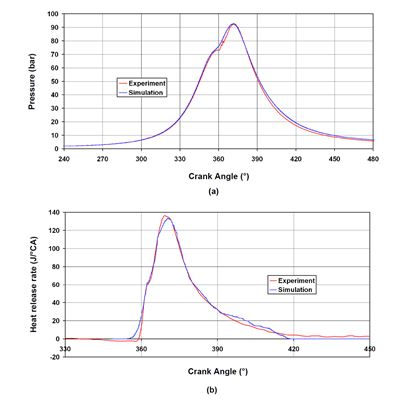Source: Authors’ original work

Figure 4. . Cylinder pressure (a) and Heat release rate (b) for operating point 5 (2399 rpm; 153.19 kW)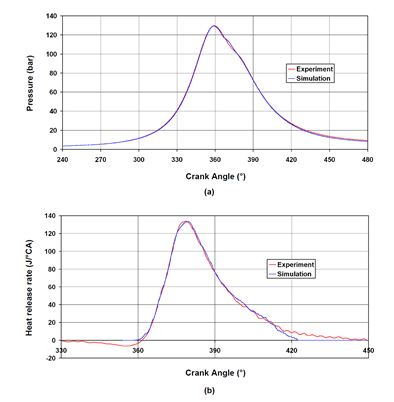Source: Authors’ original work

Raptotasios investigated the large marine two-stroke slow speed diesel engine with multizone (quasi-dimensional) combustion model (Raptotasios et al., 2015). The authors have noted that their multizone combustion model, when compared to the measurements, has maximum relative errors of 3.3%, 0.6% and 2.4% for brake specific fuel consumption, peak firing pressure and peak compression pressure. The presented relative errors refer to the engine low-load, while for the other loads they are below 0.5%. The authors have observed a higher relative error (up to 6%) for the turbine inlet temperature.

The quasi-dimensional model presented in this paper is also tested on the large marine two-stroke slow speed diesel engine (Mrzljak et al., 2016b) . The comparison of the model and the measurements at engine low-load show that this QD model has maximum relative errors of 2.1%, 4.3% and 2% for brake specific fuel consumption, peak firing pressure and peak compression pressure. The relative errors mentioned refer to the engine low-load while for the highest loads they are below 1%. For the turbine inlet temperature, the relative errors lower than 1.6% for the entire engine load range are observed. A parameter which deviates up to 9.5% is intake manifold temperature (the reasons for such deviations are described in detail). This QD model must be further improved for more precise simulations of large marine two-stroke slow speed diesel engines.

##### 5. RESULTS OF NUMERICAL MODELS AND DISCUSSION

The developed quasi-dimensional model with all of its sub-models was tested in all engine operating points presented in Table 2 and in all operating points numerical model and the experiment showed a good agreement of the results. The spray packages penetration and the gas inflow from the ZWC are shown for operating point 2, Table 2. The numerical model results are presented for one selected fuel spray and for randomly selected fuel spray packages with different axial indexes i and radial indexes j. Each of the analyzed internal combustion engine cylinders has one fuel injector with seven nozzle holes. Each nozzle hole has the same diameter, so in the developed quasi-dimensional numerical model all the fuel sprays are observed as the identical ones.

The spray package penetration from the fuel injector nozzle for three selected packages from the first axial row is presented in Figure 5. As expected, during the same time interval (crank angle), a spray package located at the fuel spray’s edge (j = 5) has the shortest penetration. In the package, the gas from ZWC inflows the earliest, which is reflected in the greatest decrease of the package penetration. A package in the fuel spray core (j = 1) has the longest penetration in the same time interval because the gas from the ZWC inflows in that package the latest.

Figure 5. The first axial row packages penetration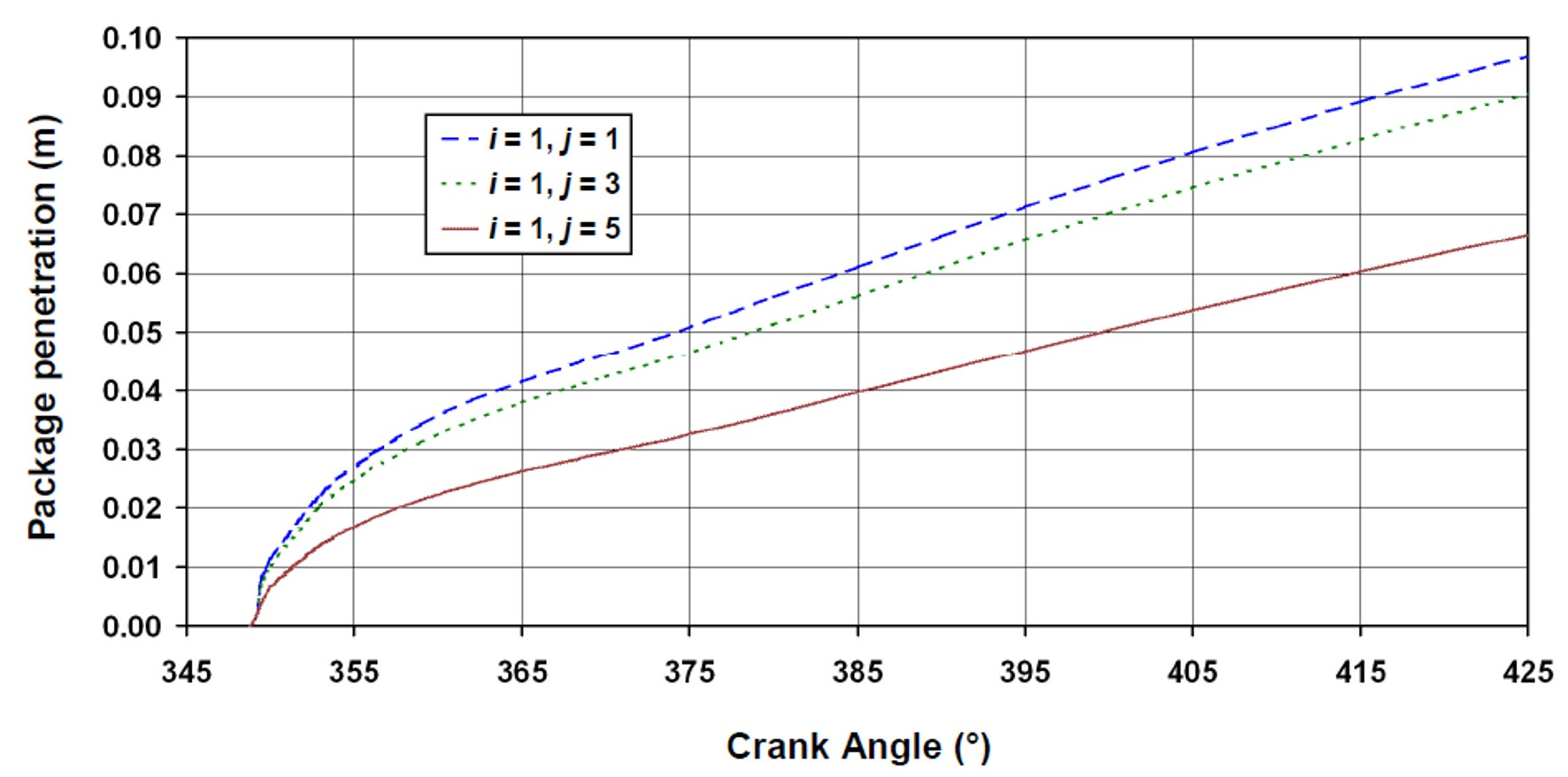Source: Authors’ original work

The same conclusion from Figure 5 is also valid for Figure 6 or for any observed spray packages from the same axial row, but with different radial index j. The shape of the curves for penetrations is the only observed difference for the fourth fuel spray axial row in comparison with the first axial row. From Figure 5 and Figure 6 we can see that before reaching the break-up time, equation (5), each spray package penetration is linear. After reaching the break-up time, the package penetration is no more linear, it has a curved form, according to equation (8).

The engine cylinder current geometry defines maximum spray package penetration. Therefore, at a certain moment, the fuel spray package will hit into the cylinder walls or in the piston head. After that moment, separate equations for the calculation of the operating media characteristic for each package, depending on the current mixture composition, is used in quasi-dimensional numerical model. Figure 5 and Figure 6 present penetration for each spray package if the package travelled freely through space without impact into the cylinder walls or in the piston head, by using the engine operating parameters presented in Table 1 and Table 2 for calculation. Although the displayed curves are based on the real engine process, they also show an additional numerical modeling capability, what enables its applicability to a wide range of diesel engines, not only on the selected one.

Figure 6. The fourth axial row packages penetration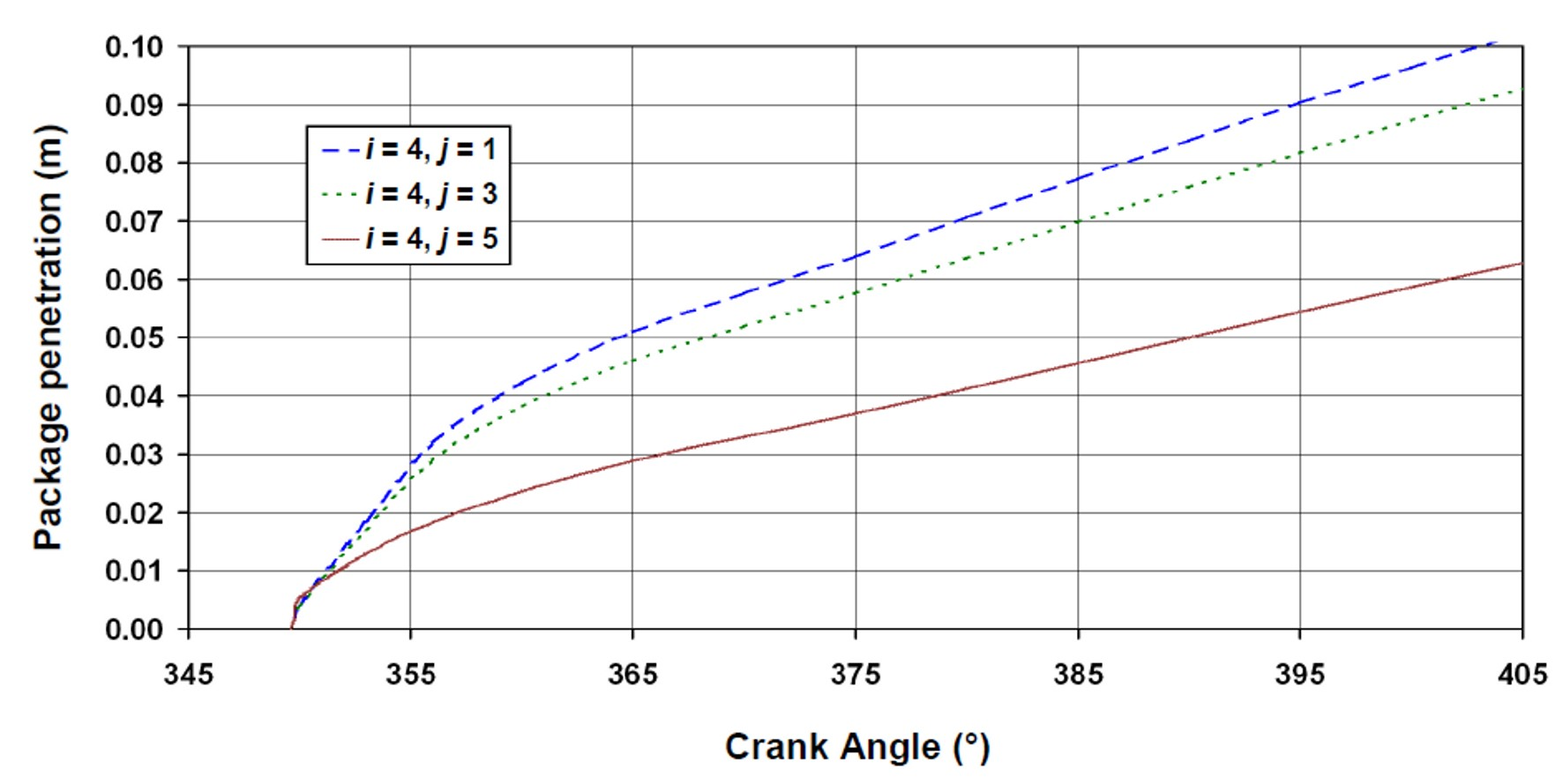Source: Authors’ original work

Figure 7 presents the later injected spray packages (packages from the tenth axial row) very quickly reach and surpass earlier injected spray packages (packages from the first axial row).

Figure 7. The first and the tenth axial row packages penetration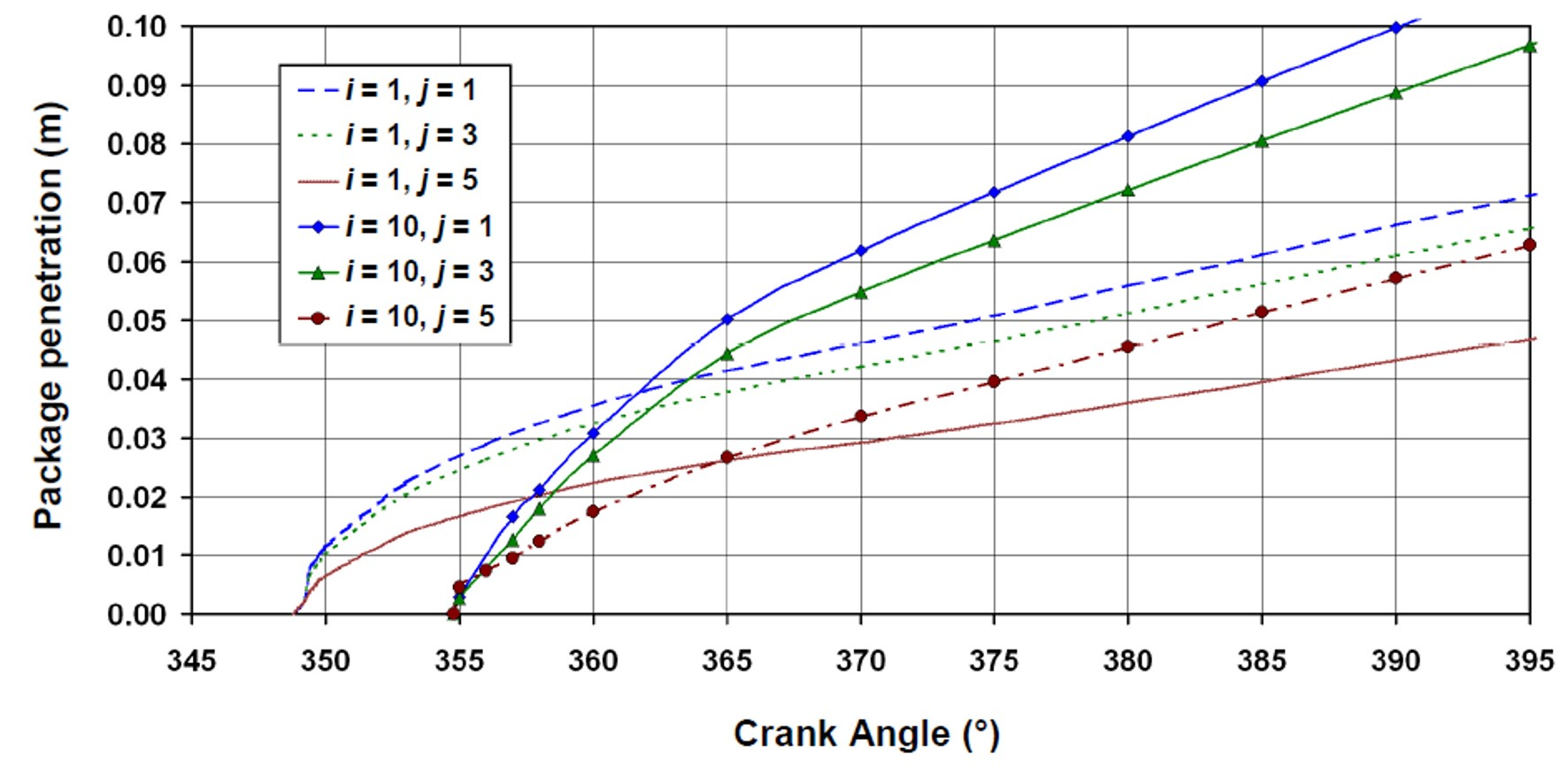Source: Authors’ original work

This occurrence is justified by the fact that packages from the first axial row, which are injected into engine cylinder the earliest, hit the so-called “gas wall” and they are more “braked” with surrounding gas than those which are injected later. Later injected spray packages travelled in the “shadow” of the front spray parts, so their penetration through the engine cylinder is more intensive. With applied correction of the coefficient B in defining the break-up time from the equation (1) and equation (2), each package penetration curve is smooth, without any unexpected occurrences.

Figure 8, Figure 9 and Figure 10 present the gas inflow into the fuel spray packages from the ZWC for different spray axial rows. In all the Figures data from the real engine process in observed operating point before and after each spray package hits into the cylinder walls or in the piston head is presented. Regardless of the spray package axial row, the gas inflow is the most intense in packages closest to the zone without combustion (packages with radial index j = 5). The gas inflow into the spray package has a lower intensity as the package is closer to the fuel spray core, so the minimum gas inflow intensity is for packages located in the fuel spray core (packages with radial index j = 1). The gas from the ZWC contains mostly fresh air with a small share of combustion products which have not been thrown out of the cylinder from the previous combustion process (combustion products which are retained in the cylinder compression volume). The amount of gas which inflows into a spray package depends on the package velocity, on the amount of liquid fuel contained in the package, on the momentum of package movement, the package distance from the fuel spray core and on the other variables described in the mathematical model. The gas inflow from the ZWC into the spray packages stops at the moment when the minimum required mass in the ZWC is reached. From that moment on, the change of the spray package volume depends only on the thermodynamic processes inside the package, not on the gas inflow from the ZWC.

The minimum required mass in the ZWC is a program-defined claim in the quasi-dimensional numerical model in order to avoid the gas inflow in spray packages which does not physically exist in the ZWC. Without that defined claim, the simulation would not follow a real physical process, which would lead to the abortion of the simulation execution.

Figure 8. Gas inflow from the ZWC into spray packages for packages from the first axial row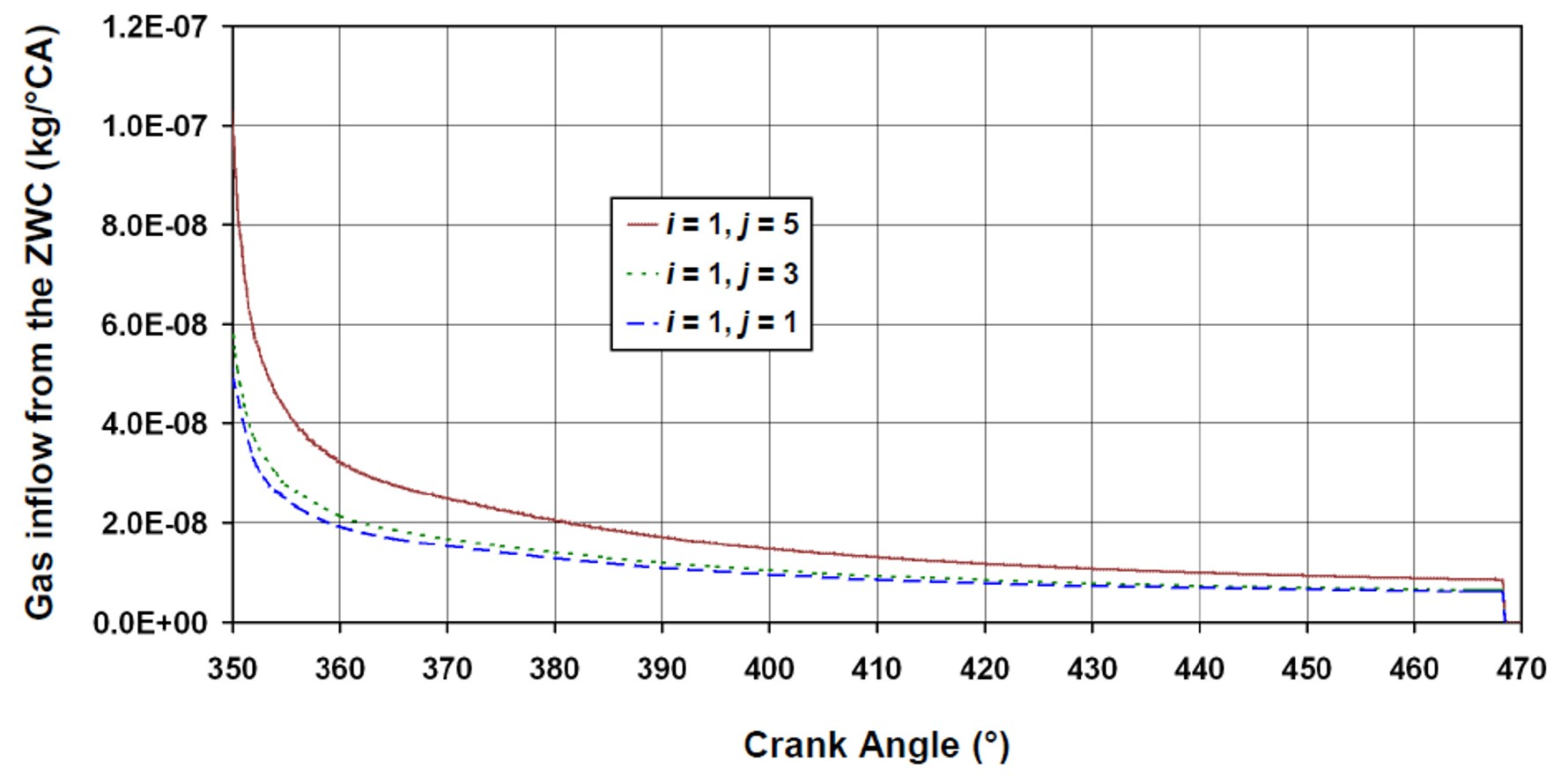Source: Authors’ original work

After the break-up time is reached, according to equation (2), the gas from the ZWC starts to inflow into each spray package. The gas inflow intensity has the same trend for any spray package. At the start of the inflow, the inflow mass is small, but it reaches maximum values very fast. After reaching maximum values it constantly decreases until the minimum mass in the ZWC is reached. A decrease from maximum inflow mass (several crank angles after an inflow begin) until reaching the minimum mass in the ZWC is shown in Figure 8, Figure 9 and Figure 10. Exactly at the inflow start the mass is small, before reaching maximum values and these small values cannot be presented on the same scale, therefore that inflow part is not displayed.

When compared, the results for packages at the fuel spray’s edge (j = 5), for different axial indexes, numerical model show that inflow mass is the lowest for the i = 1 and it amounts from 9.77E-08 kg/°CA up to 8.59E-09 kg/°CA, Figure 8. For i = 4 and the same radial index inflow mass amounts from 1.72E-07 kg/°CA up to 2.56E-08 kg/°CA, Figure 9, while for i = 10 and j = 5 the inflow mass amounts to 2.82E-07 kg/°CA up to 3.42E-08 kg/°CA, Figure 10. The first number for each axial row is the maximum inflow mass and the second number is the inflow mass at the moment of reaching the minimum mass in the ZWC.

Figure 9. Gas inflow from the ZWC into spray packages for packages from the fourth axial row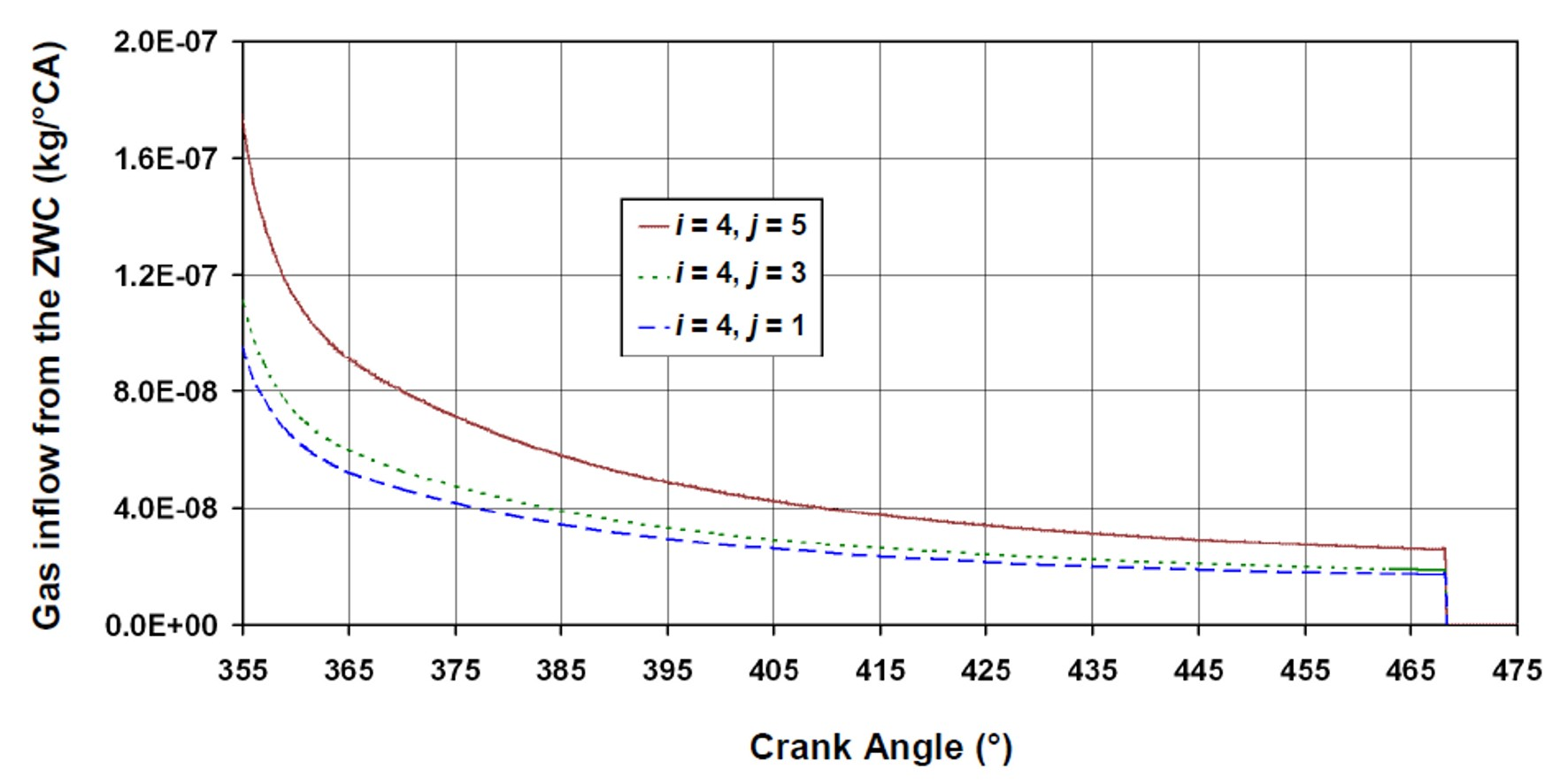Source: Authors’ original work

Figure 10. Gas inflow from the ZWC into spray packages for packages from the tenth axial row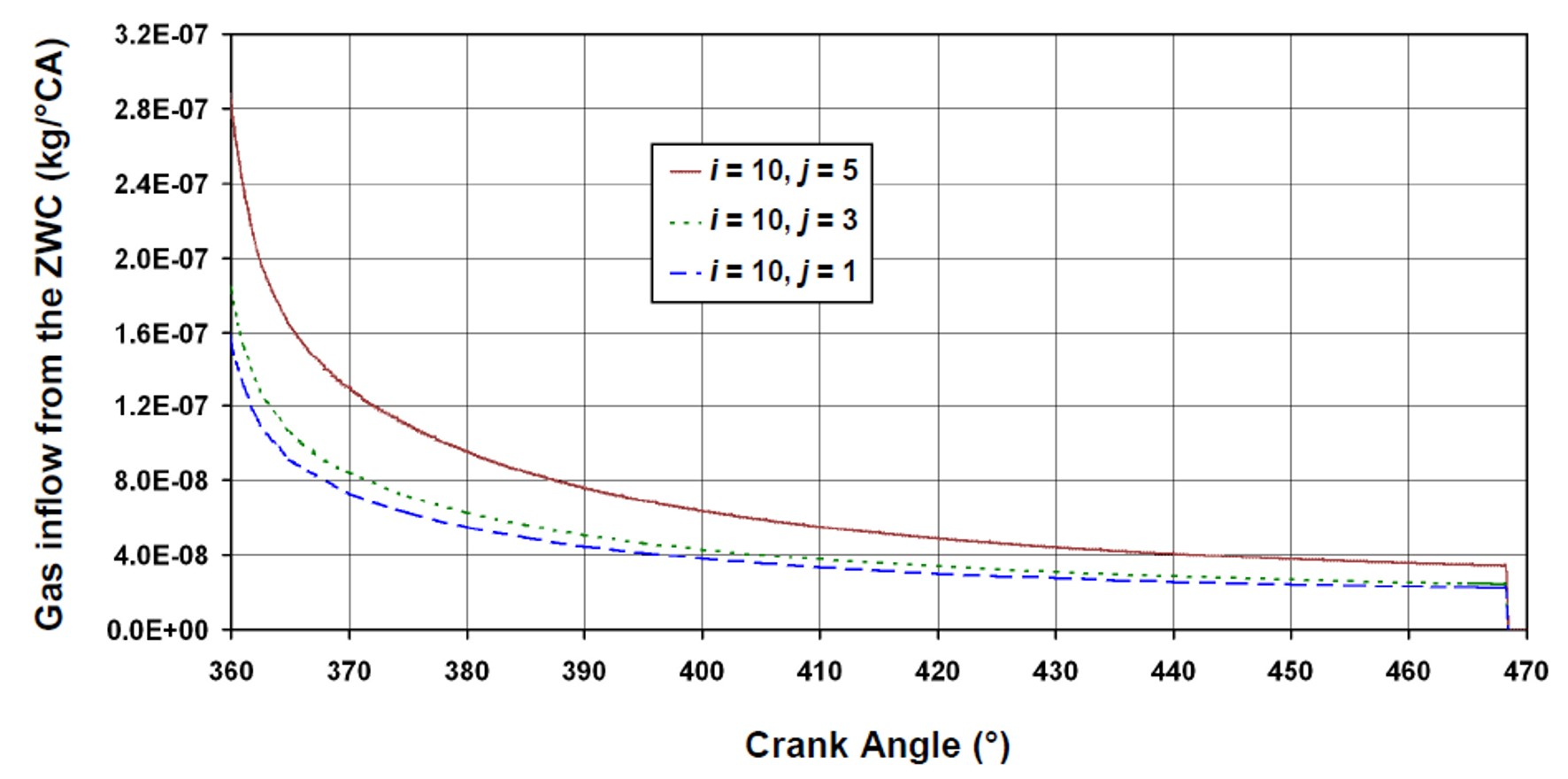Source: Authors’ original work

Therefore, it can be concluded that the inflow mass from the ZWC increases for the spray packages injected later.It should also be noted that the liquid fuel mass does not have to be the same for each spray package because the liquid fuel mass in each package depends on several fuel injection changeable variables. The same conclusions defined for the presented spray packages are also valid for all the other spray packages from the each fuel spray.

##### 6. CONCLUSIONS

The quasi-dimensional numerical models for the simulation of internal combustion engines are developed as a compromise between other known numerical models. The QD models predict engine operating parameters with appropriate accuracy and precision level along with short execution time.

This paper presents two important components of the developed quasi-dimensional numerical model: the first is a spray package penetration and the second is a gas inflow from the ZWC into the spray packages. The correction of the spray package penetration along the fuel spray radial axis in order to obtain smooth curves for each spray package is presented. The resulted spray packages penetration curves follow the physical laws from the real engine.

The measurements of the engine operating parameters in several operation points give enough data for a successful numerical model validation, after which the results of QD numerical model simulation are presented.

During the same time interval, the shortest penetration in spray packages located at the fuel spray’s edge is obtained. In these packages, the gas from the ZWC inflows the earliest which, as a consequence, produces a greater penetrations decrease in these packages. Before reaching the break-up time, each spray package penetration is linear and after the package penetration has a curved form. Spray packages injected later very quickly reach and surpass spray packages injected earlier. The spray packages injected earlier hit the so-called “gas wall” and they are more “braked” with the surrounding gas in comparison to those injected later. The spray packages injected later travelled in the “shadow” of the front spray parts and their penetration through the engine cylinder is higher.

The gas inflow from the ZWC into the spray packages is the most intensive for spray packages positioned closer to the zone without combustion. The gas inflow into the spray packages has a lower intensity as the packages are closer to the fuel spray core. Also, the simulation shows that the inflow mass from the ZWC increases for spray packages injected later.

Future investigations with the developed quasi-dimensional model will be based on the alternative diesel fuels and its blends in order to examine influences on diesel engine operating characteristics and engine emissions.

## ACKNOWLEDGMENT

The authors express their deepest thanks to the whole team at the Laboratory for Internal Combustion Engines and Electromobility (LICeM), at the Faculty of Mechanical Engineering, University of Ljubljana, Slovenia. This work is supported by the University of Rijeka (contract No. 13.09.1.1.05) and the Croatian Science Foundation-project DEcision Support SystEm for green and safe ship RouTing.

## References

1

Baumgarten C.. Mixture formation in internal combustion engines. Berlin : Heidelberg, Springer-Verlag; 2006.

2

Cvetić M.. Combustion modeling based on fuel injection flow in the diesel engine with direct injection. Belgrade : University of Belgrade; 2000.

3

Fathi M., Jahanian O., Ganji D.D., Wang S., Somers B.. 2017 Stand-alone single- and multi-zone modeling of direct injection homogeneous charge compression ignition (DI-HCCI) combustion engines. Applied Thermal Engineering. 125:1181–1190. https://doi.org/10.1016/j.applthermaleng.2017.07.123

4

Guan C., Theotokatos G., Chen H.. 2015. Analysis of two stroke marine diesel engine operation including turbocharger cut-out by using a zero-dimensional model. Energies. 8:5738–5764. https://doi.org/10.3390/en8065738

5

Hiroyasu H., Arai M.. Structures of Fuel Sprays in Diesel Engines = SAE Paper 900475. 1990.

6

Hiroyasu T., Miki M., Kamiura J., Watanabe S., Hiroyasu H.. 2002. Multi-Objective Optimization of Diesel Engine Emissions and Fuel Economy using Genetic Algorithms and Phenomenological Model. SAE Technical Paper 2002-01-2778. https://doi.org/10.4271/2002-01-2778

7

Hoseini S.S., Najafi G., Ghobadian B., Rahimi A., Yusaf T., Mamat R., Sidik N.A.C., Azmi W.H.. 2017 Effects of biodiesel fuel obtained from Salvia Macrosiphon oil (ultrasonic-assisted) on performance and emissions of diesel engine. Energy. 131(289):296–. https://doi.org/10.1016/j.energy.2017.04.150

8

Huang M., Gowdagiri S., Cesari X.M., Oehlschlaeger M.A.. 2016. Diesel engine CFD simulations: Influence of fuel variability on ignition delay. Fuel. 181:170–177. https://doi.org/10.1016/j.fuel.2016.04.137

9

Ishida M., Chen Z.L., Ueki H., Sakaguchi D.. Combustion analysis by two-zone model in a DI diesel engine. International symposium COMODIA 94. 1994

10

Jamrozik A.. 2017. The effect of the alcohol content in the fuel mixture on the performance and emissions of a direct injection diesel engine fueled with diesel-methanol and diesel-ethanol blends. Energy Conversion and Management. 148:461–476. https://doi.org/10.1016/j.enconman.2017.06.030

11

Jochim B., Korkmaz M., Pitsch H.. 2017. Scalar dissipation rate based multi-zone model for early-injected and conventional diesel engine combustion. Combustion and Flame. 175:138–154. https://doi.org/ 10.1016/j.combustflame.2016.08.003

12

Jung C., Park J., Song S.. 2015. Performance and NOx emissions of a biogas-fueled turbocharged internal combustion engine. Energy. 86:186–195. https://doi.org/10.1016/j.energy.2015.03.122

13

Lee S., Kim T.J.. 2017. Performance and emission characteristics of a DI diesel engine operated with diesel/DEE blended fuel. Applied Thermal Engineering. 121:454–461. https://doi.org/10.1016/j.applthermaleng.2017.04.112

14

Liu J., Sun P., Huang H., Meng J., Yao X.. 2017 Experimental investigation on performance, combustion and emission characteristics of a common-rail diesel engine fueled with polyoxymethylene dimethyl ethers-diesel blends. Applied Energy. 202:527–536. https://doi.org/10.1016/j.apenergy.2017.05.166

15

Mayer W.O.H., Branam R.. 2004. Atomization characteristics on the surface of a round liquid jet. Experiments in Fluids. 36(4):528–839. https://doi.org/10.1007/s00348-003-0675-0

16

Medica V.. Simulation of turbocharged diesel engine driving electrical generator under dynamic working conditions. Rijeka : University of Rijeka; 1988.

17

Mehta P.S., Bhaskar T.. Prediction of Combustion and In-Cylinder Emissions in a Direct Injection Diesel Engine Using Multi-Process Models. COMODIA 2001, The Fifth International Symposium on Diagnostics and Modeling of Combustion in Internal Combustion Engines, Nagoya. 2001. https://doi.org/10.1299/jmsesdm.01.204.14

18

Meloni R., Naso V.. 2013. An insight into the effect of advanced injection strategies on pollutant emissions of a heavy-duty diesel engine. Energies. 6():4331–4351. https://doi.org/10.3390/en6094331

19

Mrzljak V.. Quasi-dimensional model for numerical simulations of marine two-stroke diesel engine. Rijeka : University of Rijeka; 2015.

20

Mrzljak V., Medica V., Mrakovčić T.. 2015. Simulation of diesel engine cylinder process using quasi-dimensional numerical model. Scientific Journal of Maritime Research. 29:165–169

21

Mrzljak V., Medica V., Bukovac O.. 2016a Volume agglomeration process in quasi-dimensional direct injection diesel engine numerical model. Energy. 115:658–667. https://doi.org/10.1016/j.energy.2016.09.055

22

Mrzljak V., Medica V., Bukovac O.. 2016b Simulation of a Two-Stroke Slow Speed Diesel Engine Using a Quasi-Dimensional Model. Transactions of Famena. 2:35–44. https://doi.org/10.21278/TOF.40203

23

Mrzljak V., Medica V., Bukovac O.. 2017. Quasi-Dimensional diesel engine model with direct calculation of cylinder temperature and pressure. Technical Gazette. 24(3):681–686. https://doi.org/10.17559/TV-20151116115801

24

Othman M.F., Adam A., Najafi G., Mamat R.. 2017 Green fuel as alternative fuel for diesel engine: A review. Renewable and Sustainable Energy Reviews. 80:694–709. https://doi.org/10.1016/j.rser.2017.05.140

25

Rajak U., Nashine P., Singh T.S., Verma T.N.. 2018 Numerical investigation of performance, combustion and emission characteristics of various biofuels. Energy Conversion and Management. 156:235–252. https://doi.org/10.1016/j.enconman.2017.11.017

26

Rakopoulos C.D., Rakopoulos D.C., Giakoumis E.G., Kyritsis D.C.. 2004. Validation and sensitivity analysis of a two zone Diesel engine model for combustion and emissions prediction. Energy Conversion and Management. 45:1417–1495. https://doi.org/10.1016/j.enconman.2003.09.012

27

Ramesh N., Mallikarjuna J.M.. 2017. Low Temperature Combustion Strategy in an Off-Highway Diesel Engine – Experimental and CFD study. Applied Thermal Engineering. 124:844–854. https://doi.org/10.1016/j.applthermaleng.2017.06.078

28

Raptotasios S.I., Sakellaridis N.F., Papagiannakis R.G., Hountalas D.T.. 2015 Application of a multi-zone combustion model to investigate the NOx reduction potential of two-stroke marine diesel engines using EGR. Applied Energy. 157:814–823. https://doi.org/doi:10.1016/j.apenergy.2014.12.041

29

Senčić T.. Analisys of soot and NOx emissions reduction possibilities on modern low speed, two stroke diesel engines. Rijeka: University of Rijeka; 2010.

30

Stiesch G.. Phänomenologisches Multizonen-Modell der Verbrennung und Schadstoffbildung im Dieselmotor. Hannover: 1999.

31

Stiesch G. , author. Modeling engine spray and combustion processes. Berlin: Heidelberg, Springer-Verlag.; 2003.

32

Tamilselvan P., Nallusamy N., Rajkumar S.. 2017 A comprehensive review on performance, combustion and emission characteristics of biodiesel fuelled diesel engines. Renewable and Sustainable Energy Reviews. 79:1134–1159. https://doi.org/10.1016/j.rser.2017.05.176

33

Von Kuensberg Sarre C., Song-Charng K., Reitz D., Reitz R.. Modeling the effects of injector nozzle geometry on diesel sprays = SAE paper 1999-01-0912. 1999. https://doi.org/10.4271/1999-01-0912

34

Wang G., Yu W., Li X., Su Y., Yang R., Wu W.. 2019. Experimental and numerical study on the influence of intake swirl on fuel spray and in-cylinder combustion characteristics on large bore diesel engine. Fuel. 237:209–221. https://doi.org/10.1016/j.fuel.2018.09.156

35

Yang Z., Zhang F., Wang L., Wang K., Zhang D.. 2018. Effects of injection mode on the mixture formation and combustion performance of the hydrogen internal combustion engine,. Energy. 147:715–728. https://doi.org/10.1016/j.energy.2018.01.068

36

Zhang Z.E.J., Deng Y., Pham M., Zuo W., Peng Q., Yin Z.. 2018. Effects of fatty acid methyl esters proportion on combustion and emission characteristics of a biodiesel fueled marine diesel engine. Energy Conversion and Management. 159:244–253. doi:10.1016/j.enconman.2017.12.098

## Appendices

### NOMENCLATURE

 Abbreviations Latin symbols 0D Zero-Dimensional d fuel injector nozzle diameter, m ANN Artificial Neural Networks i axial fuel spray package index BMEP Brake Mean Effective Pressure j radial fuel spray package index CA Crank Angle k fuel spray index CFD Computational Fluid Dynamics m mass, kg CO Carbon Monoxide n engine speed, rpm DI Direct Injection RS swirl coefficient HC Hydro-Carbons S spray package penetration, m NOx Nitrogen Oxides t time, s PM Particulate Matter vi fuel injection velocity, m/s QD Quasi-Dimensional vp spray package current velocity, m/s ZWC Zone Without Combustion Subscripts Greek symbols b break-up Δp pressure difference, Pa corr correction ρ density, kg/m3 f fuel g gas (from the ZWC) gi gas inflow in initial max maximum min minimum

Posjeta: 284 *